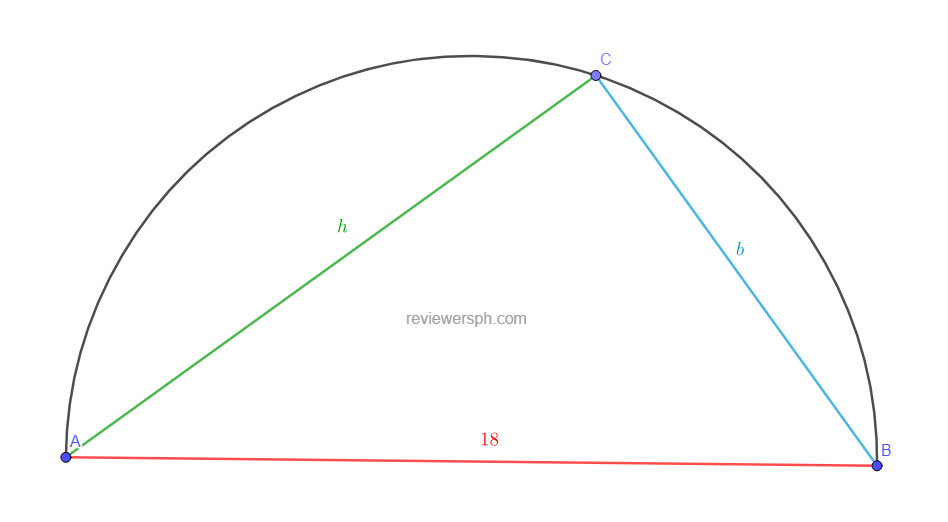### Math Notes

Subjects

#### Plane Geometry Solutions

##### Topics || Problems

A circle having a radius of 9 cm circumscribes a right triangle whose area is 43.23 sq. cm. If one of the sides is 18 cm long, find the other sides.
Solution:The area of a triangle, $$A_t = \frac{1}{2} bh$$

$$A_t = 43.23 = \frac{1}{2} bh$$

$$b =\frac{86.46}{h}$$

By Phytagorean Theorem: $$18^2 = b^2 +h^2$$

$$18^2 = (\frac{86.46}{h})^2 + h^2$$

$$324h^2= 86.46^2 + h^4$$

Let $$h^4 = a^2$$

$$0 = a^2 -324a + 86.46^2$$

$$(a-162)^2 = 162^2-86.46^2$$

$$a = 298.999$$

$$h = \sqrt{a} = 17.29$$

$$b = \frac{86.46}{17.29} = 5$$

The other sides are 5 cm and 17.29 cm